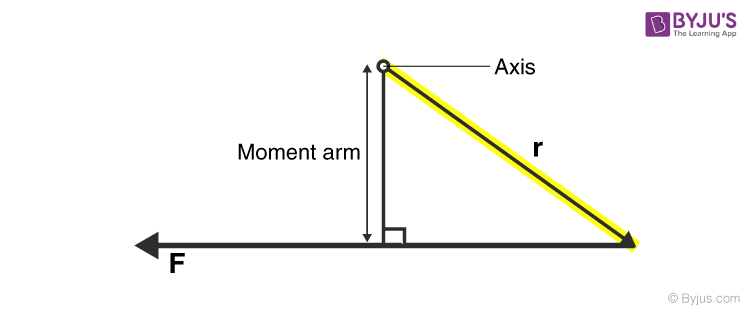# Rigid Body Dynamics and Rigid Body

In order to describe the attitude of a rigid body and to determine its evolution as a function of its initial angular velocity and applied torques, Euler’s angles and Euler’s equations of motion need to be introduced. The transformation matrix between different reference frames will be recalled.

A rigid body is an idealization of a solid body where the deformations occurring on the body are neglected. In other words, the distance between any two given points of a rigid body remains a constant regardless of the external force acting upon it.

The concept of Rigid body and Rigid body dynamics was developed to solve a range of problems that could not be explained with classical physics. Motions such as rotation of a fan, a potter’s wheel, a top, etc cannot be adequately explained with a point mass. In real life, in the case of bodies such as wheels and steel rods, deformation or bending is considered to be negligible, and we treat them as rigid.

### Rigid Body Dynamics:The question is, what kind of motion can a rigid body have? This new outlook meant that there now could be two different types of rigid body motion. We could explore and understand more kinds of problems and in doing so develop a deeper understanding of the world around us. Rigid Body Dynamics laid the foundation to what has come after, Quantum Mechanics. The two types of motion a rigid body can undergo are;

• Translational Motion
• Rotational Motion

### Configuration Space for a Rigid Body:

A macroscopic body is made up of a very large number of atoms. Describing the motion of such a system without some simplifications is clearly impossible. Many objects of interest, however, are very well approximated by the assumption that the distances between the atoms in the body are fixed. This constitutes a set of holonomic constraints, but not independent ones, as we have here $$\frac{1}{2}n(n−1)$$ constraints on 3n coordinates.

Rather than trying to solve the constraints, we can understand what are the generalized coordinates by recognizing that the possible motions which leave the interparticle lengths fixed are combinations of translations of the body as a whole, $$\vec{r}_{\alpha }\rightarrow \vec{r}_{\alpha }+\vec{C}$$ and rotations of the body about some fixed, or “marked”, point.

We will need to discuss how to represent the latter part of the configuration, (including what a rotation is), and how to re-express the kinetic and potential energies in terms of this configuration space and its velocities.

The first part of the configuration, describing the translation, can be specified by giving the coordinates of the marked point fixed in the body, $$\tilde{R}(t)$$. Often, but not always, we will choose this marked point to be the center of mass $$\vec{R}(t)$$ of the body.

In order to discuss other points that are part of the body, we will use an orthonormal coordinate system fixed in the body, known as the body coordinates, with the origin at the fixed point $$\tilde{R}$$.

The constraints mean that the position of each particle of the body has fixed coordinates in terms of this coordinate system. Thus, the dynamical configuration of the body is completely specified by giving the orientation of these coordinate axes in addition to $$\tilde{R}$$. This orientation needs to be described relative to a fixed inertial coordinate system, or inertial coordinates, with an orthonormal basis $$\tilde{e}_{i}$$.

Stay tuned with BYJU’S to learn more about Rigid Body Dynamics, Translational Motion and Rotational Motion, and much more with interesting video lectures.

Test Your Knowledge On Rigid Body And Rigid Body Dynamics!